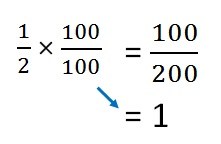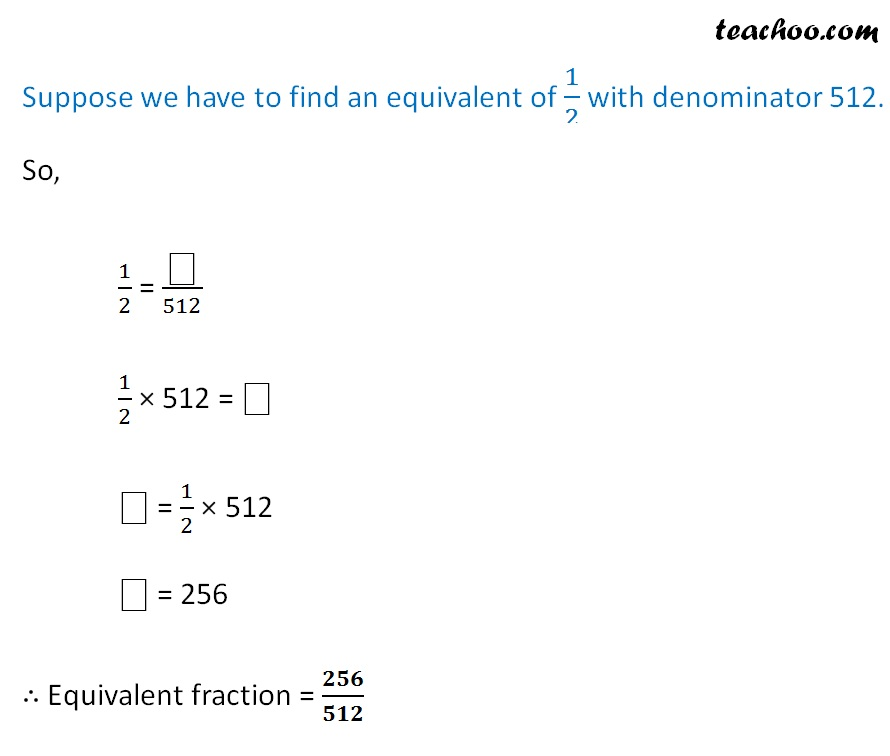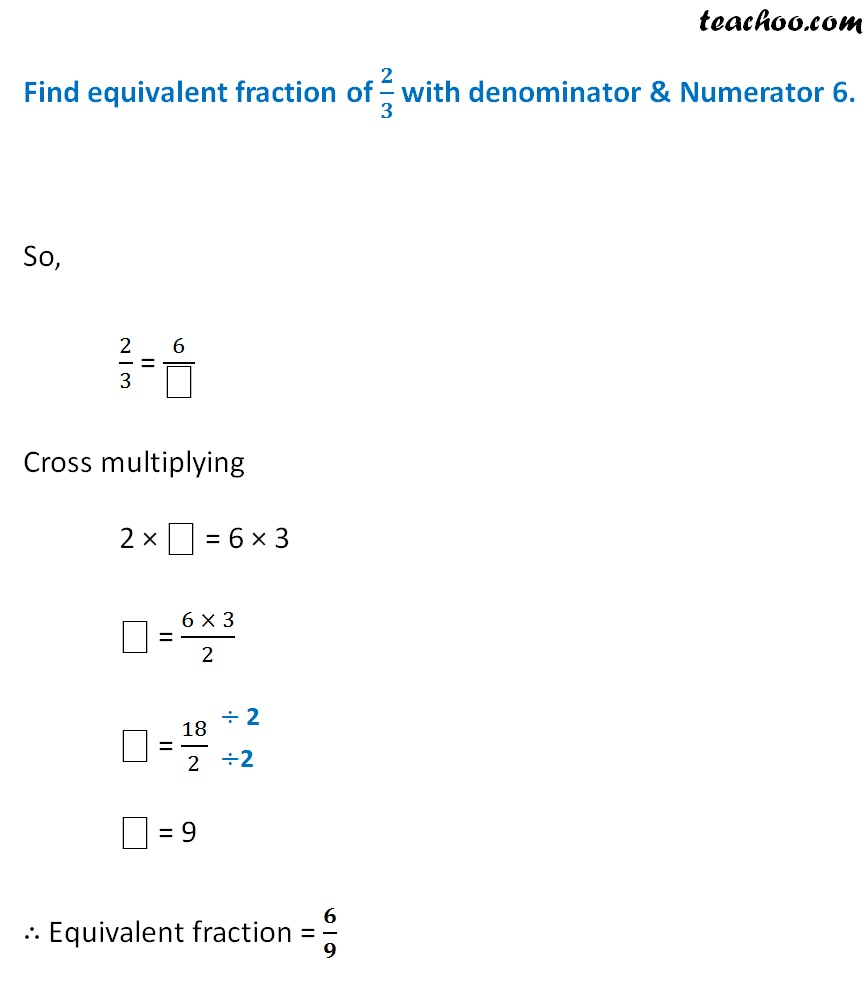Let’s take fraction

1/2

Equivalent fractions will be

1/2 × 2/2 = 2/4

1/2 × 3/3 = 3/6

1/2 × 4/4 = 4/8

1/2 × 5/5 = 5/10Here, we are multiplying by 1

So, the fraction remains the same1. Chapter 2 Class 7 Fractions and Decimals
2. Concept wise
3. Fractions

Transcript

Finding equivalent fractionsLet’s take fraction 1/2 Equivalent fractions will be 1/2 × 2/2 1/2 × 3/3 1/2 × 4/4 1/2 × 5/5 1/2 × 100/100 Here, we are multiplying by 1 So, the fraction remains the same Suppose we have to find an equivalent of 1/2 with denominator 512. So, 1/2 = "⎕" /512 1/2 × 512 = "⎕" "⎕" = 1/2 × 512 "⎕" = 256 ∴ Equivalent fraction = 𝟐𝟓𝟔/𝟓𝟏𝟐 Find equivalent fraction of 𝟐/𝟑 with denominator & Numerator 6. So, 2/3 = 6/"⎕" Cross multiplying 2 × "⎕" = 6 × 3 "⎕" = (6 × 3)/2 "⎕" = 18/2 "⎕" = 9 ∴ Equivalent fraction = 𝟔/𝟗

Fractions

Chapter 2 Class 7 Fractions and Decimals
Concept wise